# OzGrid

Excel useful formulae reference sheet

Category: [Excel]  Demo Available

# Useful formulae reference sheet

The most important functions include:

 =SUM() Adds a series of cells together.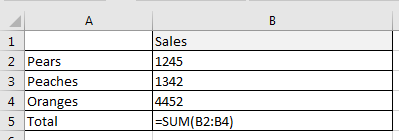=AVERAGE() Calculates the average of a series of cells.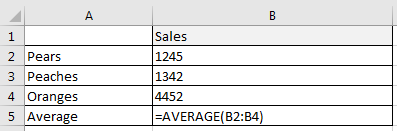=IF() checks if a condition is met and returns a value if YES and a value if NO =MIN() Provides the minimum value in a series.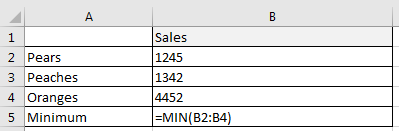=MAX() Provides the maximum value in a series.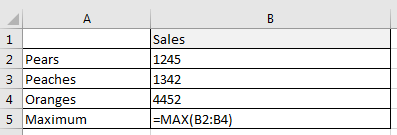=LARGE() Returns the k-th largest value in a series.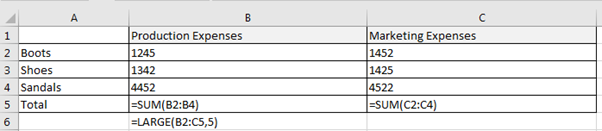=SMALL() returns the k-th smallest value in a series =COUNT() counts the number of cells in a range that contain numbers =VLOOKUP() looks for a value in the leftmost column of a table and returns in the same row from a column you specify

Free Training Course: Lesson 1 - Excel Fundamentals

Microsoft ® and Microsoft Excel ® are registered trademarks of Microsoft Corporation. OzGrid is in no way associated with Microsoft.

Click here to visit our Free 24/7 Excel/VBA Help Forum where there are thousands of posts you can get information from, or you can join the Forum and post your own questions.

### Gallery

stars (0 Reviews)